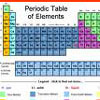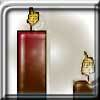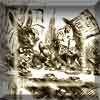# Resources tagged with: Linear equations

Filter by: Content type:
Age range:
Challenge level:

### There are 14 results

Broad Topics > Algebraic expressions, equations and formulae > Linear equations### Letter Land

##### Age 11 to 14 Challenge Level:

If: A + C = A; F x D = F; B - G = G; A + H = E; B / H = G; E - G = F and A-H represent the numbers from 0 to 7 Find the values of A, B, C, D, E, F and H.### Leonardo's Problem

##### Age 14 to 18 Challenge Level:

A, B & C own a half, a third and a sixth of a coin collection. Each grab some coins, return some, then share equally what they had put back, finishing with their own share. How rich are they?### Fibs

##### Age 11 to 14 Challenge Level:

The well known Fibonacci sequence is 1 ,1, 2, 3, 5, 8, 13, 21.... How many Fibonacci sequences can you find containing the number 196 as one of the terms?### Seven Up

##### Age 11 to 14 Challenge Level:

The number 27 is special because it is three times the sum of its digits 27 = 3 (2 + 7). Find some two digit numbers that are SEVEN times the sum of their digits (seven-up numbers)?### Good Work If You Can Get It

##### Age 11 to 14 Challenge Level:

A job needs three men but in fact six people do it. When it is finished they are all paid the same. How much was paid in total, and much does each man get if the money is shared as Fred suggests?### A Method of Defining Coefficients in the Equations of Chemical Reactions

##### Age 14 to 18

A simple method of defining the coefficients in the equations of chemical reactions with the help of a system of linear algebraic equations.### How Many Miles to Go?

##### Age 11 to 14 Challenge Level:

How many more miles must the car travel before the numbers on the milometer and the trip meter contain the same digits in the same order?##### Age 11 to 14 Challenge Level:

Think of a number and follow my instructions. Tell me your answer, and I'll tell you what you started with! Can you explain how I know?### Tweedle Dum and Tweedle Dee

##### Age 11 to 14 Challenge Level:

Two brothers were left some money, amounting to an exact number of pounds, to divide between them. DEE undertook the division. "But your heap is larger than mine!" cried DUM...### Fair Shares?

##### Age 14 to 16 Challenge Level:

A mother wants to share a sum of money by giving each of her children in turn a lump sum plus a fraction of the remainder. How can she do this in order to share the money out equally?### Terminology

##### Age 14 to 16 Challenge Level:

Given an equilateral triangle inside an isosceles triangle, can you find a relationship between the angles?### Temperature

##### Age 11 to 14 Challenge Level:

Is there a temperature at which Celsius and Fahrenheit readings are the same?### Burning Down

##### Age 14 to 16 Challenge Level:

One night two candles were lit. Can you work out how long each candle was originally?### A Problem of Time

##### Age 14 to 16 Challenge Level:

Consider a watch face which has identical hands and identical marks for the hours. It is opposite to a mirror. When is the time as read direct and in the mirror exactly the same between 6 and 7?